# Solutions of Sources of Energy (Page No- 157) - Physics By Lakhmir Singh, Class 10 Class 10 Notes | EduRev

## Class 10 : Solutions of Sources of Energy (Page No- 157) - Physics By Lakhmir Singh, Class 10 Class 10 Notes | EduRev

The document Solutions of Sources of Energy (Page No- 157) - Physics By Lakhmir Singh, Class 10 Class 10 Notes | EduRev is a part of the Class 10 Course Class 10 Physics Solutions By Lakhmir Singh & Manjit Kaur.
All you need of Class 10 at this link: Class 10

Lakhmir Singh Physics Class 10 Solutions Page No:157

Question 9: Where, in a nuclear power station, is uranium, used up ?

Solution : Uranium is used up in the reactor.

Question 10: State one use of nuclear fission reactions.

Solution : Nuclear fission reactions are used for generating electricity at a nuclear power plant.

Question 11: Name the unit which is commonly used for expressing the energy released in nuclear reactions.

Solution : Million electron volt (MeV)

Question 12: How many MeV are equivalent to 1 atomic mass unit (u) ?

Solution : 1 atomic mass unit=931 MeV

Question 13: Fill in the following blanks with suitable words :
(a) Splitting of a heavy nucleus into two lighter nuclei is called…………
(b) Uranium-235 atoms will split when hit by……… This is called……………….
(c) Nuclear………. is used in nuclear power stations for the production of electricity.
(d) In a nuclear power station, nuclear fission takes place in the…………

Solution : (a) nuclear fission
(b) neutrons; nuclear fission
(c) fission
(d) reactor

Question 14: What is nuclear fission ? Explain with an example. Write the equation of the nuclear reaction involved.

Solution : The process in which the heavy nucleus of a radioactive atom(such as uranium) splits up into smaller nuclei when bombarded with low energy neutrons, is called nuclear fission.
E.g., When uranium-235 atoms are bombarded with slow moving neutrons, the heavy uranium nucleus breaks up to produce two medium-weighted atoms and 3 neutrons, with the emission of tremendous amount of energy.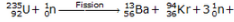tremendous amount of energy

Question 15: (a) What is nuclear fusion ? Explain with an example. Write the equation of the reaction involved.
(b) Why are very high temperatures required for fusion to occur ?

Solution : (a) The process in which two nuclei of light elements (like that of hydrogen) combine to form a heavy nucleus (like that of helium), is called nuclear fusion.
When deuterium atoms are heated to an extremely high temperature under extremely high pressure, then two deuterium nuclei combine together to form a heavy nucleus of helium, and a neutron is emitted. A tremendous amount of energy is liberated in the process.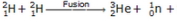tremendous amount of energy

(b) Because very high energy is required to force the lighter nuclei (which repel each other) to fuse together to form a bigger nuclei.

Question 16: What is the nuclear fuel in the sun ? Describe the process by which energy is released in the sun. Write the equation of the nuclear reaction involved.

Solution : The nuclear fuel in the sun is hydrogen gas.
The sun can be considered as a big thermonuclear furnace where hydrogen atoms continuously get fused into helium atoms. Mass gets lost during these fusion reactions and energy is being produced.
Nuclear reaction: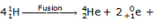tremendous amount of energy

Question 17: (a) Write Einstein’s mass-energy equation. Give the meaning of each symbol which occurs in it.
(b) If 25 atomic mass units (u) of a radioactive material are destroyed in a nuclear reaction, how much energy is released in MeV ?

Solution : (a) Einstein’s equation: E=mc2
where E is the amount of energy produced if mass m is destroyed, and c is the speed of light in vacuum.
(b) 1 atomic mass unit = 931 MeV
25 atomic mass unit = 931 x 25 MeV = 23275 MeV
23275 MeV of energy is released.

Question 18: (a) What is the source of energy of ths sun and other stars ?
(b) Describe the working of a hydrogen bomb.
(c) What is common between the sun and a hydrogen bomb ?

Solution : (a) Nuclear fusion reactions 0f hydrogen.
(b) The hydrogen bomb consists of heavy isotopes of hydrogen (deuterium and tritium) alongwith lithium-6. The explosion of hydrogen bomb is done by using an atom bomb. When the aton bomb is exploded, its fission reaction produces a lot of heat which raises the temperature of deuterium and tritium to 107oC in a few microseconds. Then fusion reactions of deuterium and tritium take place producing a tremendous amount of energy. This explodes the hydrogen bomb. Lithium-6 is used to produce more tritium needed for fusion.
(c) The source of energy is same for both the sun and the hydrogen atom, i.e. nuclear fusion.

Question 19: (a) What will happen if slow moving neutrons are made to strike the atoms of a heavy element 92U235 ? What
is the name of this process ?
(b) Write a nuclear equation to represent the process which takes place.
(c) Name one installation where such a process is utilised.

Solution : (a) When slow moving neutrons are made to strike the atoms of a heavy element uranium-235, the heavy uranium nucleus breaks up to produce two medium-weighted atoms and 3 neutrons, with the emission of tremendous amount of energy. This process is called nuclear fission.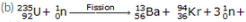tremendous amount of energy

(c) Nuclear Power Station

Question 20: (a) What are the advantages of nuclear energy ?
(b) State the disadvantages of nuclear energy.

Solution : (a) Advantages of nuclear energy:
(i) It produces a large amount of useful energy from a very small amount of a nuclear fuel.
(ii) Once the nuclear fuel is loaded into the reactor, the nuclear power plant can go on producing electricity for two to three years at a stretch. There is no need of feeding the fuel again and again.
(iii) It does not produce gases like CO2 or SO2.
(i) The waste products of nuclear fission reactions are radioactive which keep on emitting harmful radiations for thousands of years and are difficult to store or dispose safely.
(ii) Very high cost of installation is required.
(iii) There is a limited availability of uranium fuel.

Question 21: The following questions are about the nuclear reactor of a power plant.
(a) Which isotope of uranium produces the energy in the fuel rods ?
(b) Will the fuel rods last for ever ?
(c) Is the energy produced by nuclear fission or nuclear fusion ?
(d) What is the purpose of using the graphite moderator ?
(e) What is the function of boron rods in the nuclear reactor ?
(f) Why is liquid sodium (or carbon dioxide gas) pumped through the reactor ?

Solution : (a) Uranium-235
(b) No
(c) Nuclear fission
(d) Moderator slows down the speed of neutrons to make them fit for causing fission.
(e) Boron rods are used to absorb excess neutrons and prevent the fission reaction from going out of control.
(f) Liquid sodium or carbon dioxide gas is used as a ‘coolant’ to transfer the heat produced to heat exchanger for converting water into steam.

Question 22: In the reactor of a nuclear power plant, name the material which is used :
(a) as a moderator
(c) in the fuel rods
(d) in the control rods
(e) to carry away heat

Solution : (a) Graphite
(b) Concrete
(c) Uranium-235
(d) Boron
(e) Liquid sodium

Question 23: In the nuclear reactor of a power plant:
(a) how do control rods control the rate of fission ?
(b) how is heat removed from the reactor core, and what use is made of this heat ?

Solution : (a) Control rods control the rate of fission by absorbing the excess neutrons and preventing the fission reaction from going out of control.
(b) Heat is removed from the nuclear reactor core with the help of liquid sodium, which absorbs the heat and transfers it to the heat exhanger. This heat is used for converting water in the heat exchanger into steam, which is then used to produce electricity by rotating a turbine and its shaft connected to a generator.

Question 24: How does inserting the control rods in the graphite core affect the fission in the reactor ?

Solution : On inserting the control rods in the graphite core, the rods start absorbing the excess neutrons and maintain the rate of reaction as per requirement. The rods can be raised or lowered in the reactor from outside. The part which is inside the reactor absorbs neutrons.

Question 25: What are the advantages and disadvantages of using nuclear fuel for generating electricity ?

Solution : Advantages of using nuclear fuel: Electricity can be produced for almost two to three years with the same uranium fuel in a nuclear power plant.
Disadvantages of using nuclear fuel: The nuclear wastes produced by the fission of uranium-235 during the generation of electricity are radioactive and extremely harmful.

Question 26: (a) What is a nuclear reactor ? What is the fuel used in a nuclear reactor ?
(b) With the help of a labelled diagram, describe the working of a nuclear power plant.
(c) How is the working nuclear reactor of a power plant shut down in an emergency ?
(d) Name five places in India where nuclear power plants are located.

Solution : (a) Nuclear reactor is a device designed to maintain a chain reaction producing a steady flow of neutrons generated by the fission of heavy nuclei. Uranium-235 is used as a fuel in a nuclear reactor.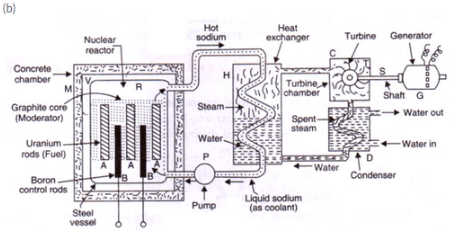In a nuclear power plant, the fission of uranium-235 is carried out in a reactor R. Uranium-235 rods are inserted in a graphite core which acts as a moderator to slow down the neutrons. Boron rods B absorb excess neutrons and controls the rate of reaction. Liquid sodium or carbon dioxide gas, which is pumped continuously through pipes embedded in reactor by using a pump P, is used as a ‘coolant’ to transfer the heat produced to heat exchanger for converting water into steam. The hot steam at high pressure goes into a turbine chamber and makes the turbine rotate. The shaft of the generator also rotates and drives a generator connected to it.
(c) By inserting the boron control rods fully into the reactor.
(d) Five places in India where nuclear powerplants are located are:
(i) Tarapur.
(ii) Kalpakkam.
(iii) Narora.
(iv) Kaprapur.
(vi) kaiga.

Offer running on EduRev: Apply code STAYHOME200 to get INR 200 off on our premium plan EduRev Infinity!

94 docs

,

,

,

,

,

,

,

,

,

,

,

,

,

,

,

,

,

,

,

,

,

,

,

,

;